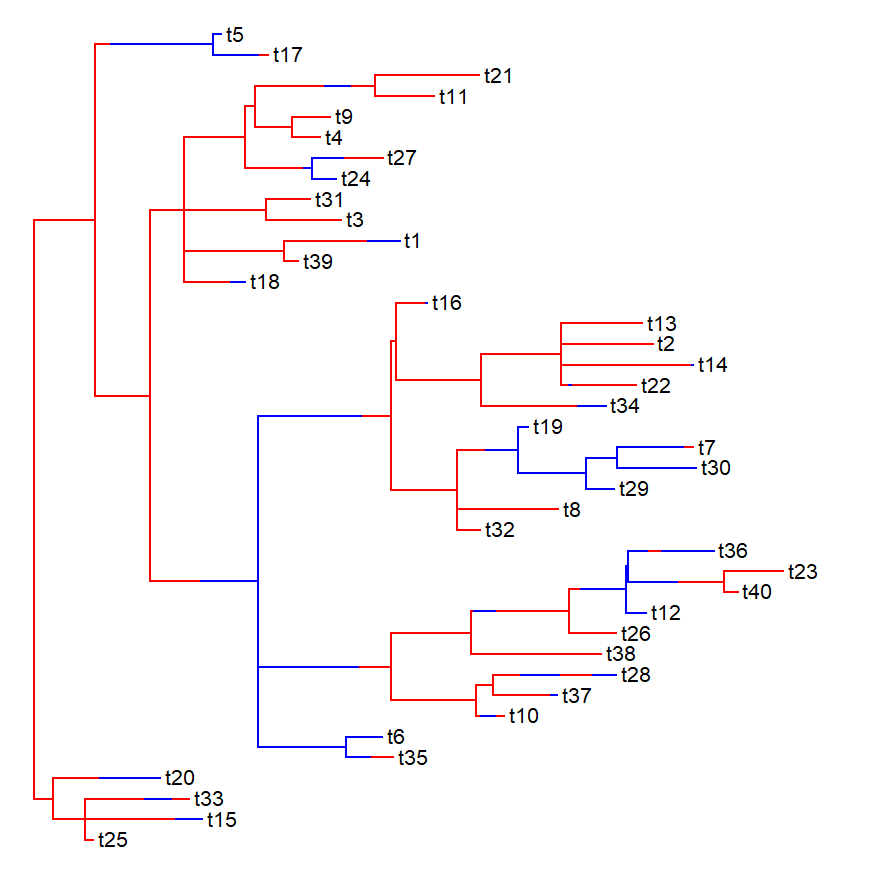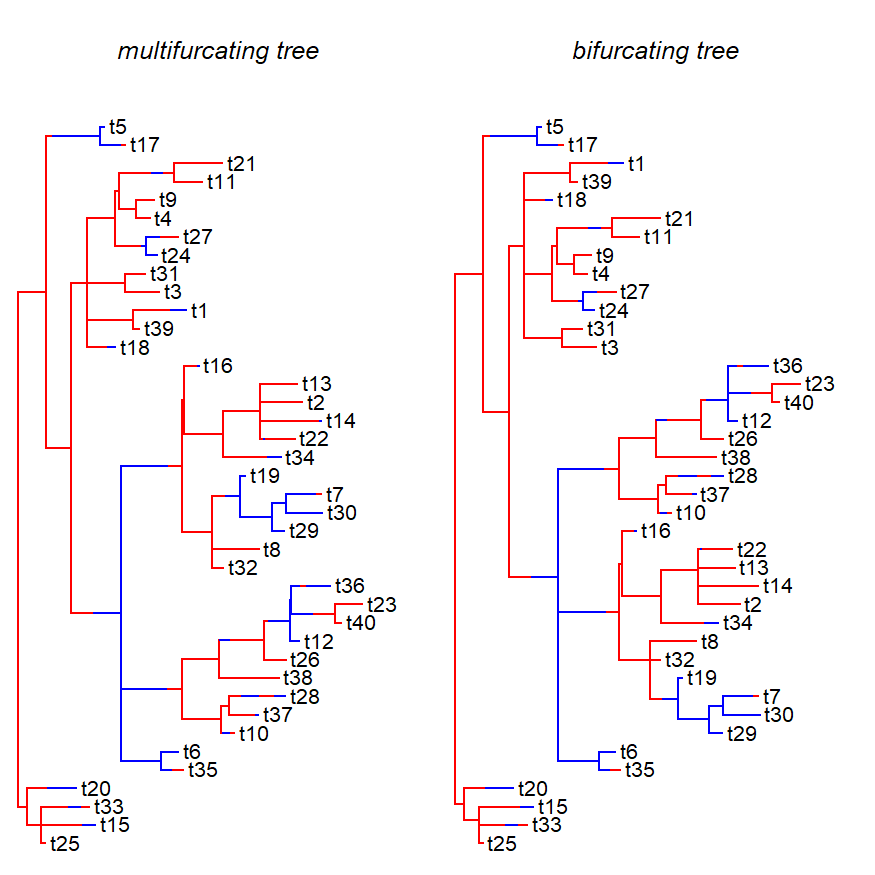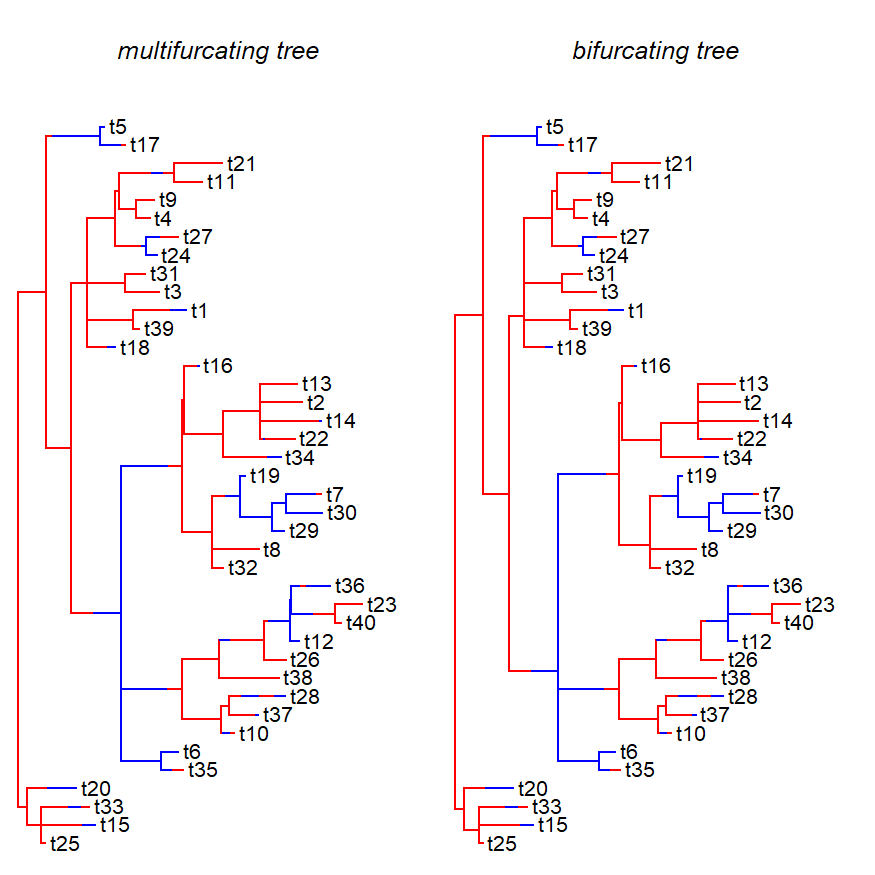## Monday, June 3, 2019

### `multi2di` method for `"simmap"` object class

I'm gradually working through alot of the functionality of phytools to see where it might be improved.

I just came across the function `di2multi.simmap`, which is registered as an S3 `di2multi` method for objects of class `"simmap"`. I discovered that there is no equivalent S3 `multi2di` method for the same object class.

The following is a pretty simple solution to this gap which uses the ape method `multi2di.phylo` internally & then just matches nodes between the two trees.

``````multi2di.simmap<-function(phy,...){
obj<-multi2di(as.phylo(phy),...)
M<-rbind(matchNodes(obj,phy),
matchLabels(obj,phy))
obj\$maps<-vector(mode="list",length=nrow(obj\$edge))
for(i in 2:nrow(M)){
if(!is.na(M[i,2])){
obj\$maps[[which(obj\$edge[,2]==M[i,1])]]<-
phy\$maps[[which(phy\$edge[,2]==M[i,2])]]
} else {
ii<-which(obj\$edge[,2]==getParent(obj,M[i,1]))
state<-names(obj\$maps[[ii]])[length(obj\$maps[[ii]])]
obj\$maps[[which(obj\$edge[,2]==M[i,1])]]<-
setNames(0,state)
}
}
obj\$node.states<-getStates(obj,"nodes")
obj\$states<-getStates(obj,"tips")
obj\$mapped.edge<-makeMappedEdge(obj\$edge,obj\$maps)
class(obj)<-c("simmap",class(obj))
obj
}
makeMappedEdge<-phytools:::makeMappedEdge ## phytools internal function
``````

It seems to work. First, let's make our multifurcating `"simmap"` object:

``````## make a multi-furcating tree:
set.seed(1)
tree<-rtree(n=40)
tree\$edge.length[which(tree\$edge[,2]==44)]<-
tree\$edge.length[which(tree\$edge[,2]==48)]<-
tree\$edge.length[which(tree\$edge[,2]==60)]<-
tree\$edge.length[which(tree\$edge[,2]==67)]<-
tree\$edge.length[which(tree\$edge[,2]==68)]<-
tree\$edge.length[which(tree\$edge[,2]==70)]<-
tree\$edge.length[which(tree\$edge[,2]==71)]<-0
tree<-di2multi(tree)
tree
``````
``````##
## Phylogenetic tree with 40 tips and 32 internal nodes.
##
## Tip labels:
##  t25, t15, t33, t20, t35, t6, ...
##
## Rooted; includes branch lengths.
``````
``````## paint a discrete character onto that tree
Q<-matrix(c(-1,1,1,-1),2,2,dimnames=list(letters[1:2],
letters[1:2]))
mtree<-sim.history(tree,Q)
``````
``````## Done simulation(s).
``````
``````cols<-setNames(c("blue","red"),c("a","b"))
plot(mtree,cols)
``````Let's resolve it & then plot the two trees together:

``````## resolve it to be dichotomous:
resolved<-multi2di(mtree)
par(mfrow=c(1,2))
plot(mtree,cols,mar=c(0.1,0.1,4.1,0.1))
title(main="multifurcating tree",font.main=3)
plot(resolved,cols,mar=c(0.1,0.1,4.1,0.1))
title(main="bifurcating tree",font.main=3)
``````We might think we can see some differences, but those are just due to the random order in which our polytomies were resolved. We can change this to `random=FALSE` & it should go away:

``````resolved<-multi2di(mtree,random=FALSE)
par(mfrow=c(1,2))
plot(mtree,cols,mar=c(0.1,0.1,4.1,0.1))
title(main="multifurcating tree",font.main=3)
plot(resolved,cols,mar=c(0.1,0.1,4.1,0.1))
title(main="bifurcating tree",font.main=3)
``````That's it! I'm going to add this to phytools now, and then also register methods for `"multiSimmap"` objects which just vectorize this & `di2multi.simmap` across a list of trees.